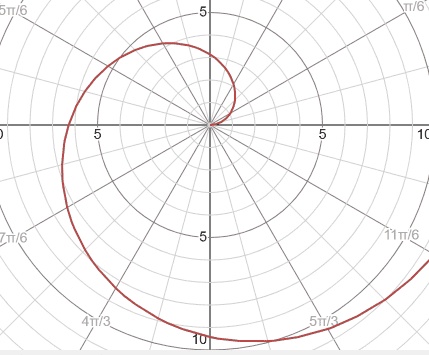# Trajectory in a non inertial frame

LCSphysicist
Homework Statement:
The hallmark of an inertial reference frame is that any object which is subject to zero net force will travel in a straight line at constant speed. To illustrate this, consider the following experiment: I am standing on the ground (which we shall take to be an inertial frame) beside a perfectly flat horizontal turntable, rotating with constant angular velocity ω. I lean over and shove a frictionless puck so that it slides across the turntable, straight through the center. The puck is subject to zero net force and, as seen from my inertial frame, travels in a straight line. Describe the puck's path as observed by someone sitting at rest on the turntable. This requires careful thought, but you should be able to get a qualitative picture. For a quantitative picture, it helps to use polar coordinates.
Relevant Equations:
r = r*ur
v = r*ur + wru(theta)
Well, make the center of the polar coordinates at the center of the turntable, so put r along the ro initial.
I am well known that to someone who is rest on the turntable, the equations will be the follow:

dr/dt = ro - vt

If the turntable route with angular velocity w,

dtheta/dt = -wt

We could go on with this and finish the question.

But i want to know about the other way that i think to resolve the question starting by the velocities:

We will have v = v = r*ur + wr*u(theta)
v = -v*ur - w(ro-vt)u(theta)

I don't know how should i proceed here..

To make it simpler, you might consider the puck to be released from the centre of the table. If the (polar) coordinates of the puck in the lab frame are ##(r, \theta) = (vt, 0)##, then what are the polar coordinates of the puck in the rotating frame? It might help to draw a quick sketch at some time ##t##.

Once you have time parameterised ##r## and ##\theta##, you can then eliminate ##t##. What sort of shape do you get?

LCSphysicist
To make it simpler, you might consider the puck to be released from the centre of the table. If the (polar) coordinates of the puck in the lab frame are ##(r, \theta) = (vt, 0)##, then what are the polar coordinates of the puck in the rotating frame? It might help to draw a quick sketch at some time ##t##.

Once you have time parameterised ##r## and ##\theta##, you can then eliminate ##t##. What sort of shape do you get?
Just to simplify the shape

r = vt
theta = wt

r = v*theta/w

r = K*theta

A type of spiral.

Well, in my question and your example it's easier see the motion by take it directly, but i am confused if i tried to do it starting with the velocity.

the minus sign because to someone in rest at the center, he doesn't route, the stone or whatever, that starts a moving this spiral.

v = v*ur ´- wr*utheta
v = v*ur - wvt*utheta

Make sense i try to integrate this? I think not, maybe is this a dead end and have to do the first approach?

LCSphysicistYou can try a velocity approach as well. It's perhaps easiest in Cartesian coordinates. In the lab frame, the velocity might be ##\vec{v} = v \hat{x}##, or more succinctly ##v_x = v##.

You might then try and find what the components of velocity ##v_x'## and ##v_y'## are in a rotating frame (with rotating ##x'## and ##y'## axes.

But be careful: the ##\hat{x}'## and ##\hat{y}'## basis vectors are also functions of time...

Last edited by a moderator:
•LCSphysicist
•